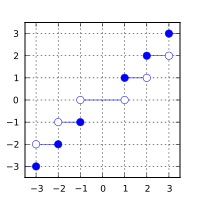/C++

# std::trunc, std::truncf, std::truncl

Defined in header `<cmath>`
```float       trunc ( float arg );
float       truncf( float arg );```
(1) (since C++11)
`double      trunc ( double arg );`
(2) (since C++11)
```long double trunc ( long double arg );
long double truncl( long double arg );```
(3) (since C++11)
`double      trunc ( IntegralType arg );`
(4) (since C++11)
1-3) Computes the nearest integer not greater in magnitude than `arg`.
4) A set of overloads or a function template accepting an argument of any integral type. Equivalent to 2) (the argument is cast to `double`).

### Parameters

 arg - floating point value

### Return value

If no errors occur, the nearest integer value not greater in magnitude than `arg` (in other words, `arg` rounded towards zero) is returned.

Return valueArgument

### Error handling

Errors are reported as specified in `math_errhandling`.

If the implementation supports IEEE floating-point arithmetic (IEC 60559),

• The current rounding mode has no effect.
• If `arg` is ±∞, it is returned, unmodified
• If `arg` is ±0, it is returned, unmodified
• If arg is NaN, NaN is returned

`FE_INEXACT` may be (but isn't required to be) raised when truncating a non-integer finite value.

The largest representable floating-point values are exact integers in all standard floating-point formats, so this function never overflows on its own; however the result may overflow any integer type (including `std::intmax_t`), when stored in an integer variable.

The implicit conversion from floating-point to integral types also rounds towards zero, but is limited to the values that can be represented by the target type.

### Example

```#include <cmath>
#include <iostream>
int main()
{
std::cout << std::fixed
<< "trunc(+2.7) = " << std::trunc(+2.7) << '\n'
<< "trunc(-2.9) = " << std::trunc(-2.9) << '\n'
<< "trunc(-0.0) = " << std::trunc(-0.0) << '\n'
<< "trunc(-Inf) = " << std::trunc(-INFINITY) << '\n';
}```

Possible output:

```trunc(+2.7) = 2.000000
trunc(-2.9) = -2.000000
trunc(-0.0) = -0.000000
trunc(-Inf) = -inf```